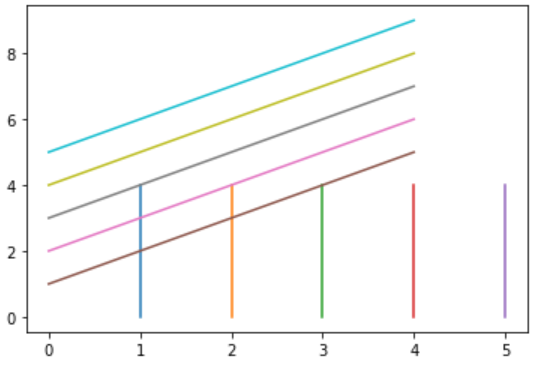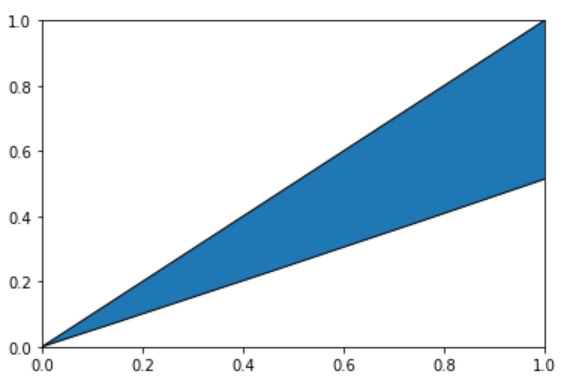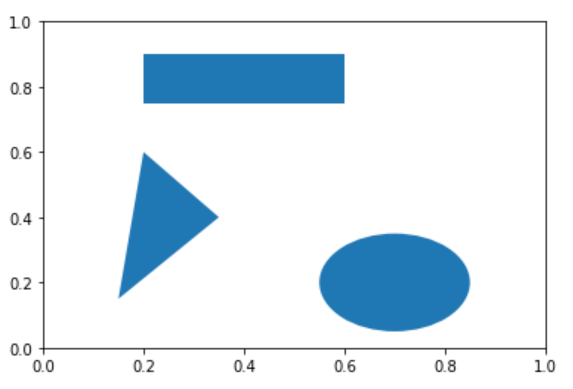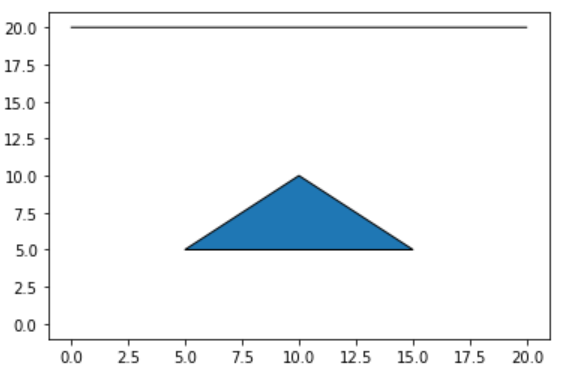# How to add a patch in a plot in Python ?

• Last Updated : 16 Mar, 2021

Matplotlib is an amazing visualization library in Python for 2D plots of arrays. Matplotlib is a multi-platform data visualization library built on NumPy arrays and designed to work with the broader SciPy stack. The Axes Class contains most of the figure elements: Axis, Tick, Line2D, Text, Polygon, etc., and sets the coordinate system. And the instances of Axes supports callbacks through a callbacks attribute. The Matplotlib.axes.Axes.add_patch() method in the axes module of matplotlib library is used to add a Patch to the axes’ patches; return the patch.

Attention geek! Strengthen your foundations with the Python Programming Foundation Course and learn the basics.

To begin with, your interview preparations Enhance your Data Structures concepts with the Python DS Course. And to begin with your Machine Learning Journey, join the Machine Learning - Basic Level Course

Parameters: This method accepts the following parameters.

• line: This parameter is the Patch to the axes’ patches.

Return value: This method returns the Patch.

Below are various examples that depict how to add a patch in a plot in Python:

Example 1:

## Python3

 `# import modules``import` `numpy as np ``import` `matplotlib.pyplot as plt `` ` `# adjust figure and assign coordinates``y, x ``=` `np.mgrid[:``5``, ``1``:``6``] ``poly_coords ``=` `[(``25``, ``75``), (``25``, ``75``), (``25``, ``75``), (``25``, ``75``)]``fig, ax ``=` `plt.subplots() `` ` `# depict illustration``cells ``=` `ax.plot(x, y, x ``+` `y) ``ax.add_patch(plt.Polygon(poly_coords)) `` ` `plt.show() `

Output:Example 2:

## Python3

 `# import modules``import` `matplotlib.path as mpath``import` `matplotlib.patches as mpatches``import` `matplotlib.pyplot as plt`` ` `Path ``=` `mpath.Path`` ` `# adjust figure and assign coordinates``fig, ax ``=` `plt.subplots()``pp ``=` `mpatches.PathPatch(Path([(``0``, ``0``), (``10``, ``5``), (``10``, ``10``), (``20``, ``10``)],``                             ``[Path.MOVETO, Path.CURVE3,``                              ``Path.CURVE3, Path.CLOSEPOLY]),``                        ``transform``=``ax.transData)`` ` `# depict illustration``ax.add_patch(pp)``plt.show()`

Output:Example 3:

## Python3

 `# import modules``import` `matplotlib.path as mpath``import` `matplotlib.patches as mpatches``import` `matplotlib.pyplot as plt`` ` `# adjust figure and assign coordinates``fig ``=` `plt.figure()``ax ``=` `fig.add_subplot(``1``, ``1``, ``1``)`` ` `pp1 ``=` `plt.Rectangle((``0.2``, ``0.75``),``                    ``0.4``, ``0.15``)`` ` `pp2 ``=` `plt.Circle((``0.7``, ``0.2``), ``0.15``)`` ` `pp3 ``=` `plt.Polygon([[``0.15``, ``0.15``],``                   ``[``0.35``, ``0.4``],``                   ``[``0.2``, ``0.6``]])`` ` `# depict illustrations``ax.add_patch(pp1)``ax.add_patch(pp2)``ax.add_patch(pp3)`

Output:Example 4:

## Python3

 `# import module``from` `matplotlib.patches ``import` `PathPatch``from` `matplotlib.path ``import` `Path``import` `matplotlib.pyplot as plt``import` `numpy as np`` ` `# assign coordinates``coord ``=` `[(``0``, ``20``), (``20``, ``20``), (``20``, ``20``),``         ``(``20``, ``20``), (``10``, ``10``), (``10``, ``10``),``         ``(``5``, ``5``), (``15``, ``5``), (``0``, ``0``)]`` ` `instn ``=` `[Path.MOVETO, Path.LINETO, Path.LINETO,``         ``Path.LINETO, Path.CLOSEPOLY, Path.MOVETO,``         ``Path.LINETO, Path.LINETO, Path.CLOSEPOLY]`` ` `# adjust figure``coord ``=` `np.array(coord, ``float``)``path ``=` `Path(coord, instn)``pathpatch ``=` `PathPatch(path)``fig, ax ``=` `plt.subplots()``ax.add_patch(pathpatch)`` ` `# depict illustration``ax.autoscale_view()``plt.show()`

Output:My Personal Notes arrow_drop_up